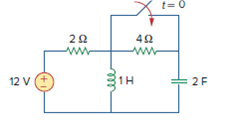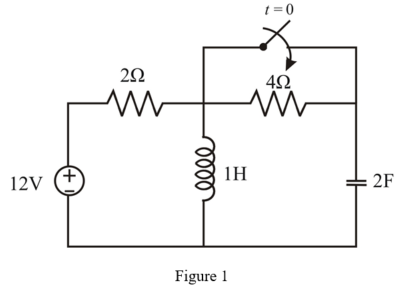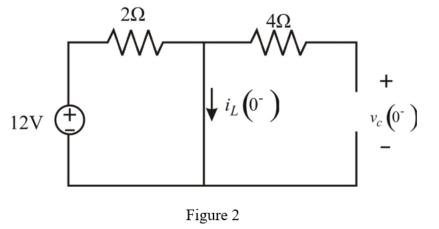Problem

# For the circuit in Fig. 8.58, the initial inductor current (at t = 0) is:(a) 0 A(b) 2 A(c)...

For the circuit in Fig. 8.58, the initial inductor current (at t = 0) is:

(a) 0 A

(b) 2 A

(c) 6 A

(d) 12 A

Figure 8.58:#### Step-by-Step Solution

Solution 1

Consider the following given circuit diagram as shown in Figure 1.Switch is closed at t = 0, before that switch is opened for a long time. So, the circuit reached to steady state and inductor becomes open circuited and capacitor becomes short circuited.

At t = 0- .

Consider the following modified circuit diagram at t = 0- as shown in Figure 2.From the circuit shown in Figure 1 , inductor is short circuited.

Therefore, inductor current cannot be zero.

Thus, option (a) is incorrect.

Apply KVL to the closed loop.

\begin{aligned} &-12+(2) i_{L}\left(0^{-}\right)=0 \\ &2 i_{L}\left(0^{-}\right)=12 \\ &i_{L}\left(0^{-}\right)=\frac{12}{2} \\ &i_{L}\left(0^{-}\right)=6 \mathrm{~A} \ldots \ldots(1) \end{aligned}

Thus, the current flowing through the inductor will be $$6 \mathrm{~A}$$ not $$2 \mathrm{~A}$$ or $$12 \mathrm{~A}$$. Thus, option (b), and (d) are also incorrect.

From equation (1), the initial inductor current $$\left(t=0^{-}\right)$$is $$6 \mathbf{A}$$. Therefore, the correct answer is option c.